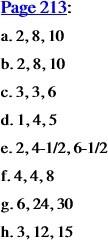Math Games & Activities, Vol. 2

09 xxxAreas of Similar Figures on the Sides of Right Triangles (pp. 213-214)

xxxxxTwo worksheets on the Pythagorean “relationship: a generalization of the Pythagorean theorem.

xxxxxThe Pythagorean theorem is a statement about right triangles, that for a right triangle with legs of lengths a and b and hypotenuse of length c, a2 + b2 = c2. But since a2 is the area of the square that could be constructed on the leg of length a, b2 the area of the square that could be constructed on the leg of length b, and c2 the area of the square that could be constructed on the hypotenuse of length c, the theorem could be stated as follows, that the sum of the areas of the squares on the legs of a right triangle equals the area of the square on the hypotenuse of the right triangle. The significance of the worksheets, then, is that they illustrate that what the Pythagorean theorem asserts about squares on the sides of a right triangle can be generalized to all figures—triangles, rectangles, and circles, to name only a few—so long as the figures are similar, that is, the same shape.

xxxxxThe answers for the worksheets are as follows:

Next > | MGA Vol. 2 | Highlights | Contents | 01 | 03 | 040 | 042 | 172 | 175 | 09 | 213 | 214 | 251 | 260 | < Back